Water Flow Sensor Flowmeter Hall Flow Sensor Water Control 1-30L/min 2.0MPa YF-S201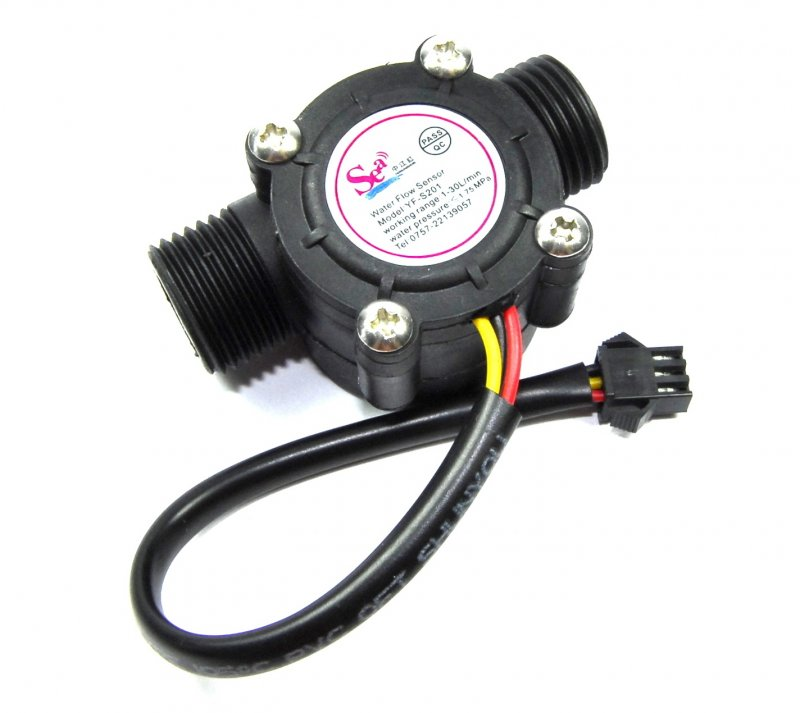Water Management System is an important part of City Management. Water management involves supplying water according to the real requirement & without wasting Water. Therefore it is very important to measure water flow rate and volume. Without measuring these parameters, Water Management is almost impossible. Also monitoring the Water Volume, Flow Rate & Water Quality remotely using Internet Connectivity has become very essential. Therefore there is a need for Monitoring Water Management System Online.42 ₪

### - Programming NodeMCU: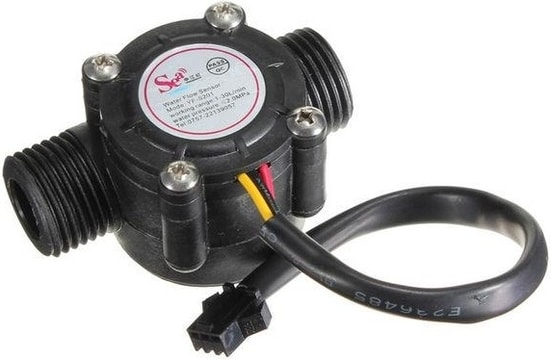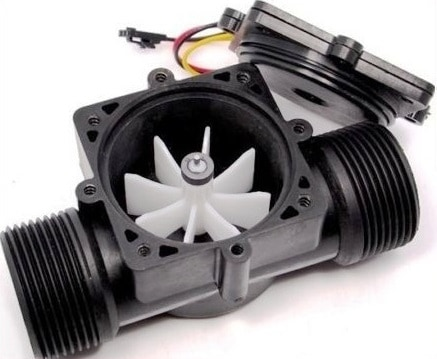# The sensor comes with three wires:

1. Red (5-24VDC power) 2. Black (ground) 3. Yellow (Hall effect pulse output)

# Work:

The water flow rate can be calculated by counting the pulses from the output of the sensor. Each pulse is approximately 2.25 milliliters. This Sensor is cheaper and best but not the accurate one as flow rate/volume varies a bit depending on the flow rate, fluid pressure, and sensor orientation. To get better precision of more than 10%, a lot of calibration is required. You can make a basic IoT Based Water Flow Meter using this Sensor. The pulse signal is a simple square wave so its quite easy to log and convert into liters per minute using the following formula.

# ESP8266 & Water Flow Sensor

Now let us interface YF-S201 Hall-Effect Water Flow Sensor with Nodemcu ESP8266 & OLED Display. The OLED Display will show Water Flow Rate & Total Volume of Water passed through the pipe. The same Flow Rate & Volume data can be sent to Thingspeak Server after an interval of 15 seconds regularly. You can switch to Blynk Application if you want immediate data. Similarly using MQTT Protocol better wireless communication can be achieved.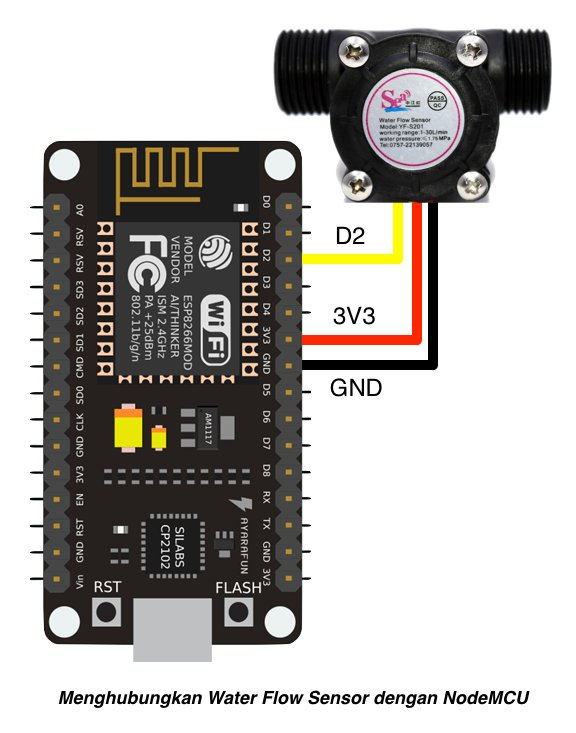we can use for NodeMCU & Arduino ---> D2 or D4 or any PIN ...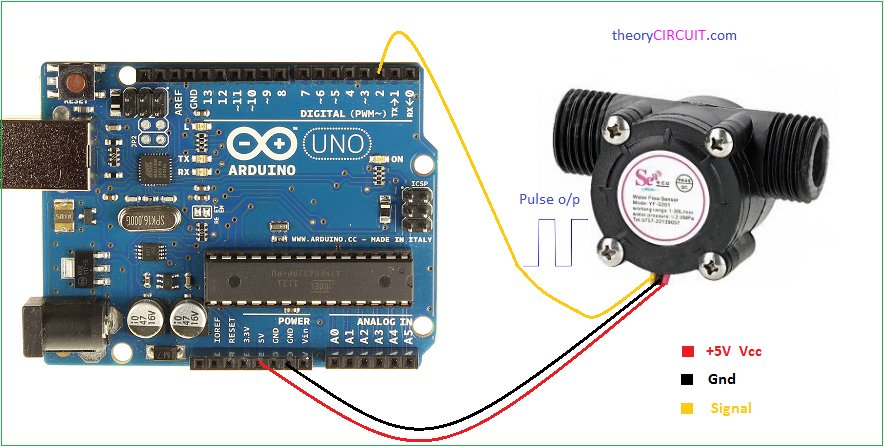# Mathematical Calculation to Measure Flow Rate & Volume

We have determined flow rate by a change in velocity of the water. The water velocity depends on the pressure that forces the through pipelines. The cross-sectional area of the pipe is known and remains constant, thus we calculate the average velocity that indicates the flow rate. Let us consider Q is the flow rate/total flow of water through the pipe, V is the average velocity & A is the cross-sectional area of the pipe. In such a case the basis relationship for determining the liquid’s flow rate in such cases is Q=VxA Sensor Frequency (Hz) = 7.5 * Q (Liters/min) Litres = Q * time elapsed (seconds) / 60 (seconds/minute) Litres = (Frequency (Pulses/second) / 7.5) * time elapsed (seconds) / 60 Litres = Pulses / (7.5 * 60)

# Circuit Diagram

The VCC (Red Wire) and GND (Black Wire) pins of flow sensors are connected to 5V and GND pins of NodeMCU while the Signal pin (Yellow Wire) is connected to D4 pin of NodeMCU.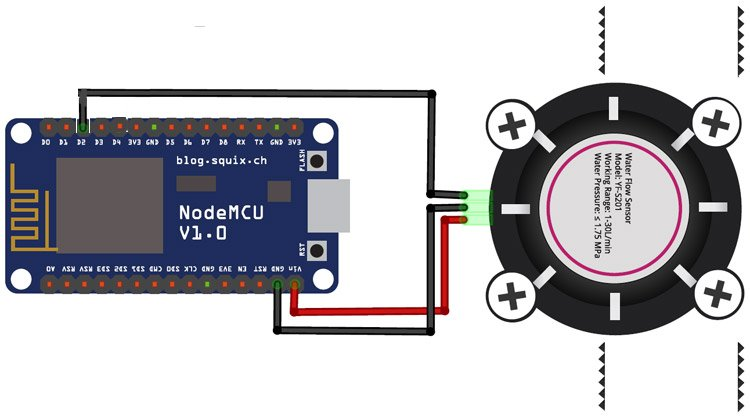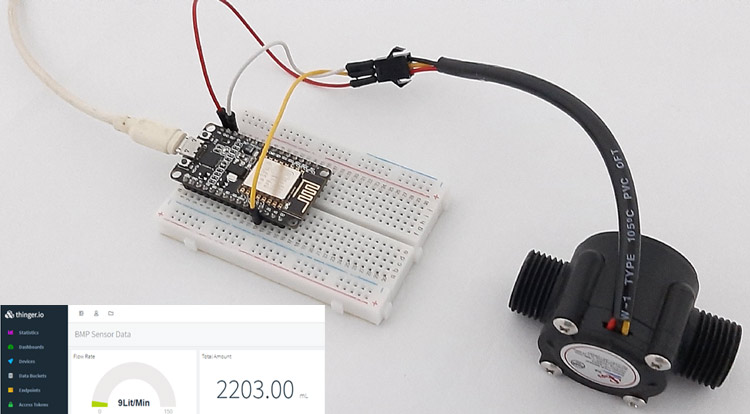# Programming NodeMCU:

we don\'t need library

code
نسخ
اقتباس
عرض
تنزيل
```
/**/

#define SENSOR  4 //D2 mean GPIO4

long currentMillis = 0;
long previousMillis = 0;
int interval = 1000;

float calibrationFactor = 4.5;
volatile byte pulseCount;
byte pulse1Sec = 0;
float flowRate;
unsigned long flowMilliLitres;
unsigned int totalMilliLitres;
float flowLitres;
float totalLitres;

void IRAM_ATTR pulseCounter()
{
pulseCount++;
}

void setup()
{
Serial.begin(115200);

pinMode(SENSOR, INPUT_PULLUP);

pulseCount = 0;
flowRate = 0.0;
flowMilliLitres = 0;
totalMilliLitres = 0;
previousMillis = 0;

attachInterrupt(digitalPinToInterrupt(SENSOR), pulseCounter, FALLING);
}

void loop()
{
currentMillis = millis();
if (currentMillis - previousMillis > interval)
{

pulse1Sec = pulseCount;
pulseCount = 0;

// Because this loop may not complete in exactly 1 second intervals we calculate
// the number of milliseconds that have passed since the last execution and use
// that to scale the output. We also apply the calibrationFactor to scale the output
// based on the number of pulses per second per units of measure (litres/minute in
// this case) coming from the sensor.
flowRate = ((1000.0 / (millis() - previousMillis)) * pulse1Sec) / calibrationFactor;
previousMillis = millis();

// Divide the flow rate in litres/minute by 60 to determine how many litres have
// passed through the sensor in this 1 second interval, then multiply by 1000 to
// convert to millilitres.
flowMilliLitres = (flowRate / 60) * 1000;
flowLitres = (flowRate / 60);

// Add the millilitres passed in this second to the cumulative total
totalMilliLitres += flowMilliLitres;
totalLitres += flowLitres;

// Print the flow rate for this second in litres / minute
Serial.print("Flow rate: ");
Serial.print(float(flowRate));  // Print the integer part of the variable
Serial.print("L/min");
Serial.print("\t");       // Print tab space

// Print the cumulative total of litres flowed since starting
Serial.print("Output Liquid Quantity: ");
Serial.print(totalMilliLitres);
Serial.print("mL / ");
Serial.print(totalLitres);
Serial.println("L");

}

}

```

code
نسخ
اقتباس
عرض
تنزيل
```
/**/

#define waterflow_pin  4 //D2 mean GPIO4

//=================================================(update_waterflow_amount)

long currentMillis = 0;
long previousMillis = 0;
int interval = 1000;//Milli second

float calibrationFactor = 4.5;
volatile byte pulseCount;
byte pulse1Sec = 0;
float flowRate;
unsigned long flowMilliLitres;
unsigned int totalMilliLitres;
float flowLitres;
float totalLitres;

void IRAM_ATTR pulseCounter()
{
pulseCount++;
}

void update_waterflow_amount()
{

currentMillis = millis();
if (currentMillis - previousMillis > interval) //each one second calculate amount
{
pulse1Sec = pulseCount;
pulseCount = 0;

// Because this loop may not complete in exactly 1 second intervals we calculate
// the number of milliseconds that have passed since the last execution and use
// that to scale the output. We also apply the calibrationFactor to scale the output
// based on the number of pulses per second per units of measure (litres/minute in
// this case) coming from the sensor.
flowRate = ((1000.0 / (millis() - previousMillis)) * pulse1Sec) / calibrationFactor;
previousMillis = millis();

// Divide the flow rate in litres/minute by 60 to determine how many litres have
// passed through the sensor in this 1 second interval, then multiply by 1000 to
// convert to millilitres.
flowMilliLitres = (flowRate / 60) * 1000;
flowLitres = (flowRate / 60);

// Add the millilitres passed in this second to the cumulative total
totalMilliLitres += flowMilliLitres;
totalLitres += flowLitres;

// Print the flow rate for this second in litres / minute
Serial.print("Flow rate: ");
Serial.print(float(flowRate));  // Print the integer part of the variable
Serial.print("L/min");
Serial.print("\t");       // Print tab space

// Print the cumulative total of litres flowed since starting
Serial.print("Output Liquid Quantity: ");
Serial.print(totalMilliLitres);
Serial.print("mL / ");
Serial.print(totalLitres);
Serial.println("L");

}

}
//=================================================(/update_waterflow_amount)

void setup()
{
Serial.begin(115200);

pinMode(waterflow_pin, INPUT_PULLUP);

pulseCount = 0;
flowRate = 0.0;
flowMilliLitres = 0;
totalMilliLitres = 0;
previousMillis = 0;

attachInterrupt(digitalPinToInterrupt(waterflow_pin), pulseCounter, FALLING);
}

void loop()
{

update_waterflow_amount();
delay(500);
}

```